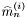#Boost C++ Libraries

...one of the most highly regarded and expertly designed C++ library projects in the world.

## Struct template kurtosis_impl

boost::accumulators::impl::kurtosis_impl — Kurtosis estimation.

## Synopsis

```// In header: <boost/accumulators/statistics/kurtosis.hpp>

template<typename Sample>
struct kurtosis_impl {
// types
typedef numeric::functional::average< Sample, Sample >::result_type result_type;

// construct/copy/destruct
kurtosis_impl(dont_care);

// public member functions
template<typename Args> result_type result(Args const &) const;
};```

## Description

The kurtosis of a sample distribution is defined as the ratio of the 4th central moment and the square of the 2nd central moment (the variance) of the samples, minus 3. The termis added in order to ensure that the normal distribution has zero kurtosis. The kurtosis can also be expressed by the simple moments:

Equation 1.2.whereare the-th moment andthe mean (first moment) of thesamples.

### `kurtosis_impl` public construct/copy/destruct

1. `kurtosis_impl(dont_care);`

### `kurtosis_impl` public member functions

1. `template<typename Args> result_type result(Args const & args) const;`